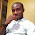## Tuesday, August 14, 2018

Cantilever slabs are common features in buildings due to the need to have bigger spaces at upper floors. To achieve this, architects normally extend the slab beyond the ground floor building line, thereby forming a cantilever. In this post, we are going to show how we can analyse and design cantilever slabs subjected to floor load and block work load.

Solved Example
A cantilever slab 200 mm thick is 1.715m long, and it is supporting a blockwork load at 1.0m from the fixed end. Design the slab using the data given below;

Purpose of building - Residential
fck = 25 Mpa
fyk = 460 Mpa
Concrete cover = 25 mm
Height of block wall = 2.75 m
Unit weight of concrete = 25 kN/m3
Unit weight of block with renderings = 3.75 kN/m2

Self weight of slab = (25 × 0.2) = 5 kN/m2
Finishes (assume) = 1.2 kN/m2
Partition allowance = 1.0 kN/m2
Total characteristic permanent action (pressure load) gk  = 7.2 kN/m2

Permanent action from wall Gk = 3.75  × 2.75 = 10.3125 kN/m

Variable action on slab qk = 1.5 kN/m2

At ultimate limit state;
n = 1.35gk + 1.5qk
n = 1.35(7.2) + 1.5(1.5) = 11.97 kN/m2

Ultimate load from wall = 1.35 x 10.3125 =  13.92 kN/m

Design Forces
MEd = (13.92 × 1) + (11.97 × 1.7152)/2 = 31.523 kNm
VEd = (13.92) + (11.97 × 1.715) = 34.45 kN

Flexural design
MEd  = 31.523 kNm
Effective depth (d) = h – Cnom – ϕ/2 - ϕlinks
Assuming ϕ12 mm bars will be employed for the main bars
d = 200 – 25 – 6 = 169 mm

k = MEd/(fckbd2) = (31.523 × 106)/(25 × 1000 × 1692) = 0.044

Since k < 0.167, no compression reinforcement required
z = d[0.5+ √(0.25 - 0.882K) ]
z = d[0.5+ √(0.25 - 0.882(0.044))] = 0.95d

As1 = MEd/(0.87fyk z) = (31.523 × 106)/(0.87 × 460 × 0.95 × 169) = 490 mm2/m
Provide X12@200 c/c  TOP (ASprov = 565 mm2/m)

Check for deflection
ρ = As,prov /bd = 565 / (1000 × 169) = 0.0033
ρ0 = reference reinforcement ratio = 10-3√(fck) = 10-3√(25) = 0.005
Since if ρ ≤ ρ0;

L/d = K [11 + 1.5√(fck) ρ0/ρ + 3.2√(fck) (ρ0 / ρ - 1)(3⁄2)

k = 0.4 (Cantilevers)

L/d = 0.4 [11 + 1.5√(25) × (0.005/0.0033) + 3.2√(25) × [(0.005 / 0.0033) - 1](3⁄2)
L/d = 0.4[11 + 11.363 + 5.9159] = 11.311

βs = (500 Asprov)/(fyk Asreq) = (500 × 565) / (460 × 490) = 1.253

Therefore limiting L/d = 1.253  × 11.311 = 14.172
Actual L/d = 1715/169 = 10.147

Since Actual L/d (10.147) < Limiting L/d (14.172), deflection is satisfactory.

Exercise for Students
(1) Provide distribution bars
(2) Verify the section for shear
(3) Check for cracking
(4) Do the detailing sketches

Thank you for visiting Structville today, and God bless.

1.why unit weight of concrete = 25kN/m3?

1.It's obvious you're not familiar with RC design to Eurocodes (BS EN 1991-1-1:2002). The bulk density of normal weight concrete(24kN/m³) which you know from BScodes was increased to account for embedded reinforcements and it's stated as 25kN/m³ for 'reinforced normal weight concrete'.

2.It`s prette much the same as we calculate in Ukraine.

3.